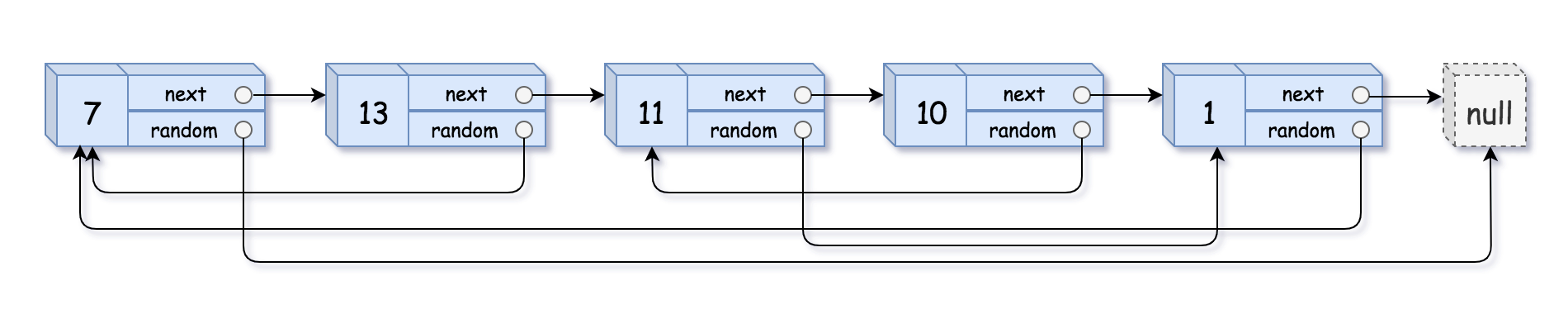# 138. Copy List with Random Pointer / Medium

A linked list of length n is given such that each node contains an additional random pointer, which could point to any node in the list, or null.

Construct a deep copy of the list. The deep copy should consist of exactly n brand new nodes, where each new node has its value set to the value of its corresponding original node. Both the next and random pointer of the new nodes should point to new nodes in the copied list such that the pointers in the original list and copied list represent the same list state. None of the pointers in the new list should point to nodes in the original list.

For example, if there are two nodes X and Y in the original list, where X.random –> Y, then for the corresponding two nodes x and y in the copied list, x.random –> y.

The linked list is represented in the input/output as a list of n nodes. Each node is represented as a pair of [val, random_index] where:

• val: an integer representing Node.val
• random_index: the index of the node (range from 0 to n-1) that the random pointer points to, or null if it does not point to any node.

## Example 1:Output: [[7,null],[13,0],[11,4],[10,2],[1,0]]

## Example 2:Output: [[1,1],[2,1]]

## Example 3:Output: [[3,null],[3,0],[3,null]]

## Constraints:

• 0 <= n <= 1000
• -10^4 <= Node.val <= 10^4
• Node.random is null or is pointing to some node in the linked list.

# Solution 1: Iterative with O(1) Space

## 思路

Solution 給出一個很有趣的做法，就是將新的 Node 接在舊的 Node 後面，這時新的 random pointer 指的就會是舊的 random pointer 的下一個。## 效能

### Complexity

• Time Complexity: O(N)
• Space Complexity: O(1)

### LeetCode Result

• Runtime: 7 ms
• Memory Usage: 11.3 MB

# Solution 2: Map

## 效能

### Complexity

• Time Complexity: O(N)
• Space Complexity: O(1)

### LeetCode Result

• Runtime: 3 ms
• Memory Usage: 11.5 MB